# Search by Topic

#### Resources tagged with Logarithmic functions similar to Catalyse That!:

Filter by: Content type:
Age range:
Challenge level:

### There are 17 results

Broad Topics > Functions and Graphs > Logarithmic functions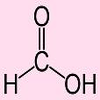### Extreme Dissociation

##### Age 16 to 18 Challenge Level:

In this question we push the pH formula to its theoretical limits.### Blood Buffers

##### Age 16 to 18 Challenge Level:

Investigate the mathematics behind blood buffers and derive the form of a titration curve.### Ph Temperature

##### Age 16 to 18 Challenge Level:

At what temperature is the pH of water exactly 7?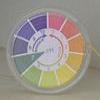### Mixing Ph

##### Age 16 to 18 Challenge Level:

Use the logarithm to work out these pH values### Drug Stabiliser

##### Age 16 to 18 Challenge Level:

How does the half-life of a drug affect the build up of medication in the body over time?### What Do Functions Do for Tiny X?

##### Age 16 to 18 Challenge Level:

Looking at small values of functions. Motivating the existence of the Taylor expansion.### Prime Counter

##### Age 16 to 18 Challenge Level:

A short challenge concerning prime numbers.### Harmonically

##### Age 16 to 18 Challenge Level:

Is it true that a large integer m can be taken such that: 1 + 1/2 + 1/3 + ... +1/m > 100 ?### Power Match

##### Age 16 to 18 Challenge Level:

Can you locate these values on this interactive logarithmic scale?### Log Attack

##### Age 16 to 18 Challenge Level:

Solve these equations.### Sierpinski Triangle

##### Age 16 to 18 Challenge Level:

What is the total area of the triangles remaining in the nth stage of constructing a Sierpinski Triangle? Work out the dimension of this fractal.### How Does Your Function Grow?

##### Age 16 to 18 Challenge Level:

Compares the size of functions f(n) for large values of n.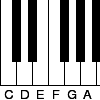### Tuning and Ratio

##### Age 16 to 18 Challenge Level:

Why is the modern piano tuned using an equal tempered scale and what has this got to do with logarithms?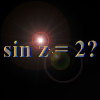### Complex Sine

##### Age 16 to 18 Challenge Level:

Solve the equation sin z = 2 for complex z. You only need the formula you are given for sin z in terms of the exponential function, and to solve a quadratic equation and use the logarithmic function.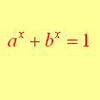### Equation Attack

##### Age 16 to 18 Challenge Level:

The equation a^x + b^x = 1 can be solved algebraically in special cases but in general it can only be solved by numerical methods.### Function Pyramids

##### Age 16 to 18 Challenge Level:

A function pyramid is a structure where each entry in the pyramid is determined by the two entries below it. Can you figure out how the pyramid is generated?### Big, Bigger, Biggest

##### Age 16 to 18 Challenge Level:

Which is the biggest and which the smallest of $2000^{2002}, 2001^{2001} \text{and } 2002^{2000}$?